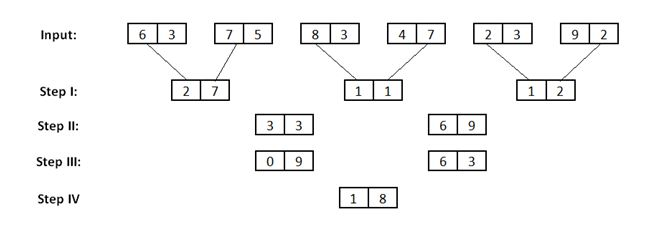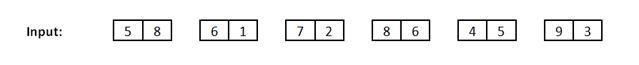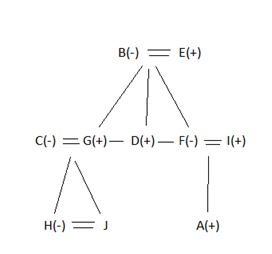# SBI Clerk Mains Reasoning (Day-14)

Dear Aspirants, Our IBPS Guide team is providing new series of Reasoning Questions for SBI Clerk Mains 2020 so the aspirants can practice it on a daily basis. These questions are framed by our skilled experts after understanding your needs thoroughly. Aspirants can practice these new series questions daily to familiarize with the exact exam pattern and make your preparation effective.

Start Quiz

Input and Output:

Directions (1 – 5): Study the given information carefully and answer the given question.

An input-output is given in different steps. Some mathematical operations are done in each step. No mathematical operation is repeated in next step but it can be repeated with some other mathematical operation (as multiplication can be used with subtraction in step 1 and same can be used with addition in step 2).As per the rules followed in the steps given above, find out in each of the following questions the appropriate step for the given input.1) What will be the final result of the given input?

a) 43

b) 88

c) 56

d) 28

e) 51

2) What is the sum of inverse of the numbers obtained in step 3?

a) 105

b) 126

c) 116

d) 106

e) None of these

3) What is the difference between the first block obtained in step 2 and second block obtained in step 3?

a) 34

b) 36

c) 54

d) 56

e) None of these

4) If the number obtained in second block of step III is multiplied by 7 what will be the resultant value?

a) 154

b) 197

c) 210

d) 147

e) 133

5) What is the resultant value, when the first digit of second block in step II is added with the square of the second digit of first block in step III?

a) 67

b) 85

c) 93

d) 77

e) 86

Blood relation:

Directions (6 – 8): Study the following information carefully and answer the questions followed:

There are ten members A, B, C, D, E, F, G, H, I and J in the family. There are three generations of the family. There is also three married couple in the family. There is an equal number of male and female. F is the daughter – in – law of E.G’s brother D have only one sister F. C and G are the married couple. C is the mother – in – law of H.B is the mother of G. I is the son in law of B. H is the sister of J. A is the only son of I.

6) If G is the son – in – law of P, then how P related to H?

a) Father

b) Grand Father

c) Grand Mother

d) Can’t be determined

e) None of these

7) If K is the son of D, then how K related to A?

a) Sister

b) Brother

c) Sister – in – law

d) Brother – in – law

e) Cousin

8) If R is the son of C, then how R related to F?

a) Son

b) Brother

c) Nephew

d) Son – in – law

e) None of these

Critical Reasoning

9) Study the following information carefully and answer the questions given below:

Statement: For the first time since starting its operations a decade and a half ago, Delhi Metro has seen a substantial dip in its daily ridership.

1. Delhi Metro has the longest connectivity network in the country.

2) Delhi Metro reaches its destination within a stipulated time frame while the buses have to face lots of traffic on the road

3) People find Delhi Metro too much crowded all along the day.

4) There has been a rise in the fare of Delhi Metro by the DMRC officials.

Which of the following will be a valid reason for the decrease in ridership by the Metro?

a) Only 1

b) Only 3

c) 3 and 4

d) 1, 2 and 3

e) None of the above

10) In the question below is given a statement followed by two courses of actions numbered I and II. A course of action is a step or administrative decision to be taken for improvement, follow-up or further action in regard to the problem, policy, etc. On the basis of the information given in the statement to be true, then decide which of the suggested courses of action logically follow(s) for pursuing.

Statement: “Air pollution caused by petrol and diesel vehicles is killing millions of people in cities around the world,” Paris Mayor Anne Hidalgo said in a statement. “The same emissions are also causing climate change.”

Courses of action:

I: The mayor should buy only zero-emission buses from 2025.

II: The mayor should promote walking, cycling and the use of public transport in Paris.

a) I

b) II

c) Either I or II

d) Neither I nor II

e) Both I and II

Directions (1 –5):

Step 1:

Difference between the product of first digits from both the blocks and product of second digits from both the blocks i.e. 6 × 7 = 42 and 3 × 5 = 15.

Difference between 42 and 15 is 27.

Same process is applied in all pair of blocks.

Step 2:

In this step, first digit of each block is added and then multiplied by 3 and the result is added to 18 i.e. (2 + 1 + 1) × 6 + 9 = 33

Same process is to be repeated (on second digits of all three blocks) to get the second block.

Step 3:

In this step, square of first digit of first block and square of first digit from second block is added and then product of first digit from both the blocks is subtracted from it i.e. 32 + 32 – 3 × 3 = 09.

Same process is done on second digits of previous blocks to get the second block of this step.

Step 4:

Difference between the sum of the blocks and difference of both the blocks is calculated to get the final answer i.e. 09 + 63 = 72; and difference between 63 and 09 is 54.

Finally, difference between 72 and 54 is 18.

Same procedure is applied for the given input:Directions (6-8) :Rise in the fare, and being too much crowded give inconvenience to riders, hence are the reasons for the decrease in ridership by the Metro.

So, option (C) is the correct answer.

Option 2 represents positive-benefit of the metro, while Option 1 illustrates a quality of the Delhi metro.